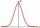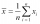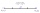# Average

The arithmetic mean of the two numbers is 71.7. One number is 5. Calculate the second number.

Result

x =  138.4

#### Solution:Leave us a comment of example and its solution (i.e. if it is still somewhat unclear...):Be the first to comment!#### To solve this verbal math problem are needed these knowledge from mathematics:

Looking for help with calculating arithmetic mean? Looking for a statistical calculator?

## Next similar examples:

1. Arithmetic averageThe arithmetic mean of the five numbers is exactly 8. The sum of these four numbers is 30. What is the fifth number?
2. AverageAverage of 7 numbers is 65. What is its sum?
3. The averageThe average of one set of 4 numbers is 35. The average of another set of number is 20. The average of the numbers in the two sets is 30. How many numbers are there in the other set?
4. Combine / add termCombine like terms 4c+c-7c
5. Median and modusRadka made 50 throws with a dice. The table saw fit individual dice's wall frequency: Wall Number: 1 2 3 4 5 6 frequency: 8 7 5 11 6 13 Calculate the modus and median of the wall numbers that Radka fell.
6. 75th percentile (quartille Q3)Find 75th percentile for 30,42,42,46,46,46,50,50,54
7. Find midpointFM=5y+13, MG=5-3y, FG=? M is the midpoint of FG. Use the given information to find the missing measure or value.
8. NormThree workers planted 3555 seedlings of tomatoes in one dey. First worked at the standard norm, the second planted 120 seedlings more and the third 135 seedlings more than the first worker. How many seedlings were standard norm?
9. BicycleThe bicycle pedal gear has 36 teeth, the rear gear wheel has 10 teeth. How many times turns rear wheel, when pedal wheel turns 120x?
10. IronIron ore contains 57% iron. How much ore is needed to produce 20 tons of iron?
11. Simple equationSolve for x: 3(x + 2) = x - 18
12. ClassIn 7.C clss are 10 girls and 20 boys. Yesterday was missing 20% of girls and 50% boys. What percentage of students missing?
13. Seeds 2How many seeds germinated from 1000 pcs, when 23% no emergence?
14. Obtuse angleWhich obtuse angle is creating clocks at 17:00?
15. Negative in equation2x + 3 + 7x = – 24, what is the value of x?
16. The percentages in practiceIf every tenth apple on the tree is rotten it can be expressed by percentages: 10% of the apples on the tree is rotten. Tell percent using the following information: a. in June rained 6 days b, increase worker pay 500 euros to 50 euros c, grabbed 21 fro
17. Passenger boatTwo-fifths of the passengers in the passenger boat were boys. 1/3 of them were girls and the rest were adult. If there were 60 passengers in the boat, how many more boys than adult were there?